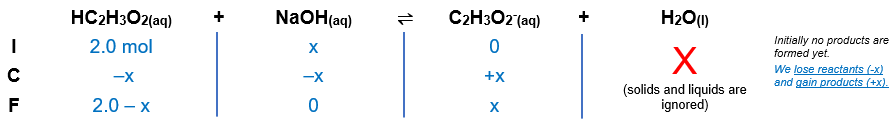# Problem: What quantity (moles) of NaOH must be added to 1.0 L of 2.0 M HC2H3O2 to produce a solution buffered at each pH?a. pH = pKa

###### FREE Expert Solution

When dealing with a buffer, we use the Henderson-Hasselbalch equation:

The reaction between HC2H3O2 and NaOH is shown below:

HC2H3O2(aq) + NaOH(aq)  C2H32-(aq) + H2O(l)
(weak acid)                       (conjugate base)

**Na+ is not included in the reaction because it is a neutral ion

Calculate the initial amount of HC2H3O2 in moles:

moles HC2H3O2 = 2.0 mol HC2H3O2

We need to construct an ICF chart to determine the amounts of the species after the reaction:Substitute values in the Henderson-Hasselbalch equation:

**log is a base of 10, when you divide a number by log, you will take 10 raised to the power of the number

96% (11 ratings)###### Problem Details

What quantity (moles) of NaOH must be added to 1.0 L of 2.0 M HC2H3O2 to produce a solution buffered at each pH?

a. pH = pKa